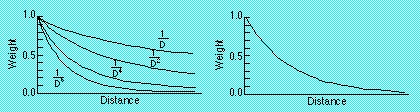## Weighting functions

The control points (or the slopes at the points) used in the estimation procedure are weighted according to the distances between the grid node being estimated and the points. In Surface III, many different weighting functions can be selected. The four weighting functions decrease at increasing rates with distance.The weights that are assigned to the control points according to the weighting function are adjusted to sum to 1.0. Therefore, the weighting function actually assigns proportional weights and expresses the relative influence of each control point. A widely used version of the weighting process assigns a function whose exact form depends upon the distance from the location being estimated and the most distant point used in the estimation. This inverse distance-squared weighting function is then scaled so that it extends from one to zero over this distance.

As in other weighting functions, the sum of the weights is set equal to 1.0.go back